## RS Aggarwal Class 6 Solutions Chapter 10 Ratio, Proportion and Unitary Method Ex 10B

These Solutions are part of RS Aggarwal Solutions Class 6. Here we have given RS Aggarwal Solutions Class 6 Chapter 10 Ratio, Proportion and Unitary Method Ex 10B.

Other Exercises

Question 1.
Solution:
(i) 4, 6, 8, 12
If it is in proportion, then
If ad = bc if 4 x 12 = 6 x 8
If 48 = 48
Which is true
∴ 4, 6, 8, 12 are in proportion
(ii) 7, 42, 13, 78
7 : 42 :: 13 : 78
If ad = bc if 7 x 78 = 42 x 13
If 546 = 546
Which is true
∴ 7, 42, 13, 78 are in proportion
(iii) 33, 121, 9, 96 or 33 : 121 :: 9 : 96
are in proportion
If 33 x 96 = 121 x 9
If 3168 = 1089
Which is not true
∴ 33, 121,9, 96 are not in proportion
(iv) 22, 33, 42, 63 or 22 : 33 :: 42 : 63
are in proportion
If 22 x 63 = 33 x 42
If 1386 = 1386
Which is true
∴ 22, 33, 42, 63 are in proportion
(v) 32, 48, 70, 210 or 32 : 48 :: 70 : 210
are in proportion
If 32 x 210 = 48 x 70
If 6720 = 3360
Which is not true
∴ 32, 48, 70, 210 are not in proportion
(vi) 150, 200, 250, 300 or
150 : 200 :: 250 : 300 are in proportion
If ad = bc if 150 x 300 = 200 x 250
If 45000 = 50000
Which is not true
∴ 150, 200,250, 300 are not in proportion

Question 2.
Solution:
(i) We have 60 : 105 :: 84 : 147
Product of means = 105 x 84 = 8820
Product of extremes = 60 x 147 = 8820
∴ Product of means = Product of extremes
Hence 60 : 105 :: 84 : 147 is verified.
(ii) We have 91 : 104 :: 119 : 136
Product of means = 104 x 119 = 12376
Product of extremes = 91 x 136 = 12376
Product of means = Product of extremes
Hence 91 : 104 :: 119 : 136 is verified.
(iii) We have 108 : 72 :: 129 : 86
Product of means = 72 x 129 = 9288
Product of extremes = 108 x 86 = 9288
Product of means = Product of extremes
Hence 108 : 72 :: 129 : 86 is verified.
(iv) We have 39 : 65 :: 141 : 235
Product of means = 65 x 141 = 9165
Product of extremes = 39 x 235 = 9165
∴ Product of means = Product of extremes
Hence 39 : 65 :: 141 : 235 is verified.

Question 3.
Solution:
(i) We have 55 : 11 :: x : 6
Product of means = 11 × x = 11x
Product of extremes = 55 x 6 = 330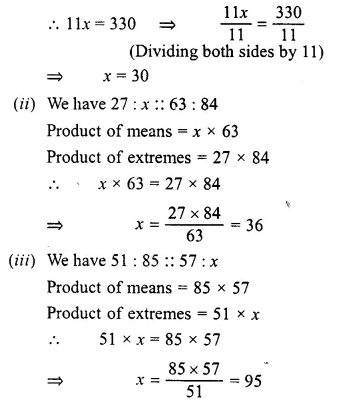Question 4.
Solution:
(i) We have, 51 : 68 = $$\\ \frac { 51 }{ 68 }$$
= $$\\ \frac { 3 }{ 4 }$$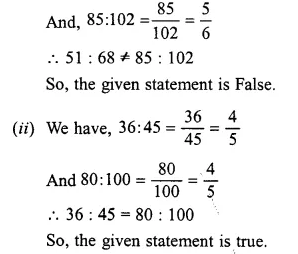Question 5.
Solution:
(i) 25 cm : 1 m and Rs. 40 : Rs. 160
= $$\\ \frac { 25cm }{ 1000cm }$$ = $$\\ \frac { 1 }{ 4 }$$,
$$\\ \frac { Rs.40 }{ Rs.160 }$$ = $$\\ \frac { 1 }{ 4 }$$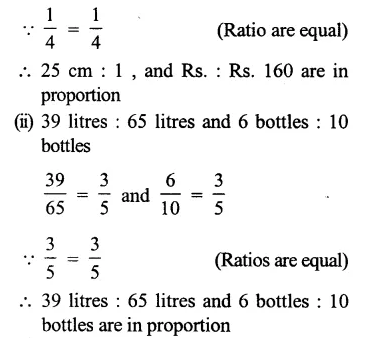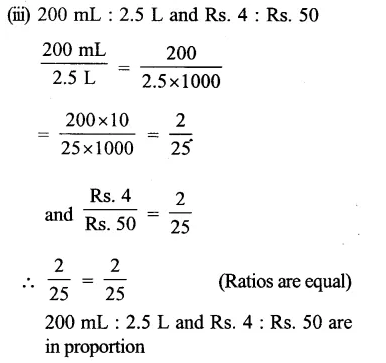Question 6.
Solution:
Let the third term be x.
Then 51 : 68 :: x : 108
Now, product of means = x × 68
And, product of extremes = 51 × 108
x × 68 = 51 × 108
=> x = $$\\ \frac { 51\times 108 }{ 68 }$$
= 3 × 27 = 81
x = 81
Hence the third term of the given proportion is 81

Question 7.
Solution:
1st term =12, third term = 8 and fourth term = 14
Let 2nd term = x, thenQuestion 8.
Solution:
(i) The given numbers 48, 60, 75 are in
continued proportion if 48 : 60 :: 60 : 75.
Now, product of means = 60 x 60 = 3600
And, product of extremes = 48 x 75 = 3600
∴ Product of means = Product of extremes
So, 48 : 60 :: 60 : 75
Hence, the numbers 48, 60, 75 are in continued proportion.
(ii) The given numbers 36, 90, 225 are in
continued proportion of 36 : 90 :: 90 : 225
Now, product of means = 90 x 90 = 8100
And, product of extremes = 36 x 225 = 8100
∴ Product of means = Product of extremes
So, 36 : 90 :: 90 : 225
Hence, the numbers 36, 90, 225 are in continued proportion.
(iii)The given numbers 16, 84, 441 are in
continued proportion if 16 : 84 :: 84 : 441.
Now, product of means = 84 x 84 = 7056
And, product of extremes = 16 x 441 = 7056
Product of means = Product of extremes.
So, 16 : 845 :: 84 : 441
Hence 16, 84, 441 are in continued proportion.
(iv) The given numbers 27, 36, 48 are
in continued proportion if 27 : 36 :: 36 : 48
Now, product of means = 36 x 36 = 1296
And, product of extremes = 27 x 48 = 1296
∴ Product of means = Product of extremes.
So, 27 : 36 :: 36 : 48
Hence, the numbers 27, 36, 48 are in continued proportional.

Question 9.
Solution:
It is given that 9, x, x, 49 are in proportion, that is, 9 : x :: x : 49
∴ Product of means = Product of extremesQuestion 10.
Solution:
Let the height of the pole be x metres.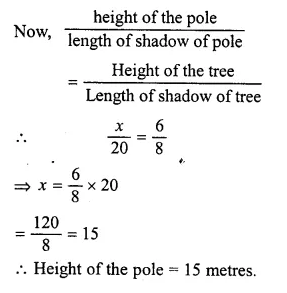Question 11.
Solution:
5 : 3 :: x : 6Hope given RS Aggarwal Solutions Class 6 Chapter 10 Ratio, Proportion and Unitary Method Ex 10B are helpful to complete your math homework.

If you have any doubts, please comment below. Learn Insta try to provide online math tutoring for you.